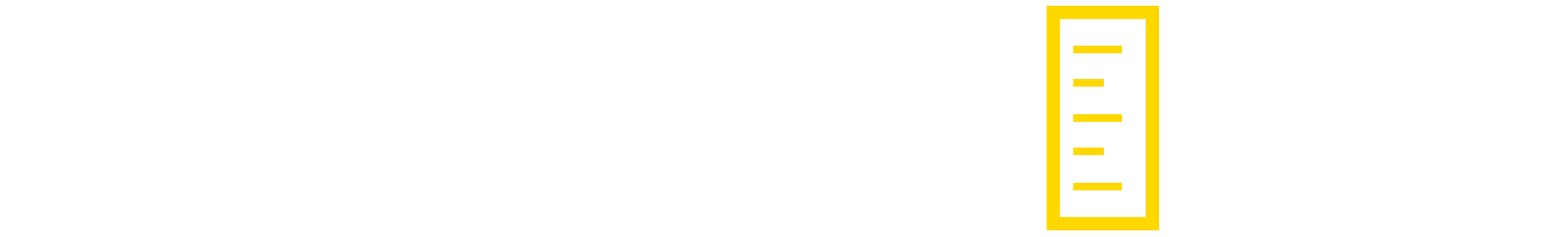# Documentation

Overview

Real Size is an editor tool that automatically extends the Transform component inspector for all game objects. No extra components are required.

Installation and Uninstallation

Import Real Size into your project.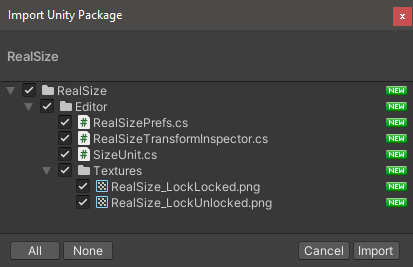Real Size adds no other dependencies to your project and can be removed at any time by deleting the “RealSize” folder within your “Assets” folder. Keep in mind that this will also remove your Real Size preferences, including any custom units you have defined.

Inspector

Transform component inspector with Real Size Collapsed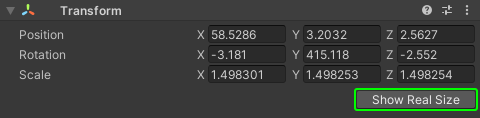When not in use, Real Size collapses to a single button in the Transform component.

Transform component inspector with Real Size Expanded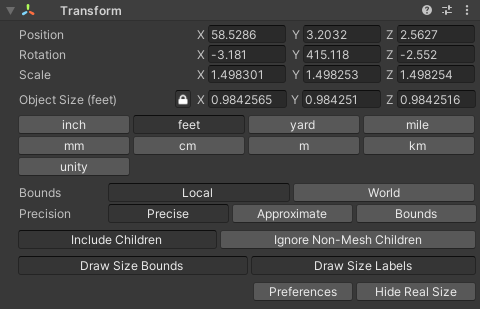Real Size default settings

Object Size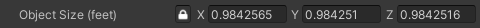The size of the bounds of the selected object using the selected size unit.

Uniform Scale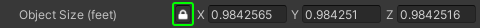With Uniform Scale enabled, modifying one axis will proportionally scale all axes.

Scaling Y Axis (unmodified)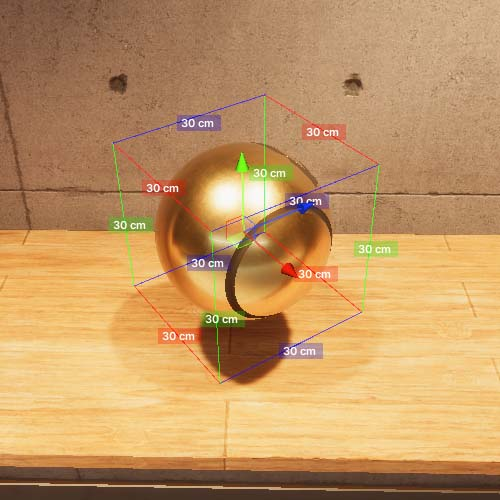Uniform Scale Enabled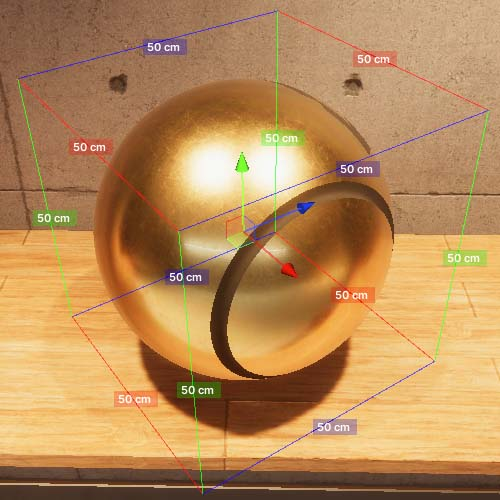Uniform Scale Disabled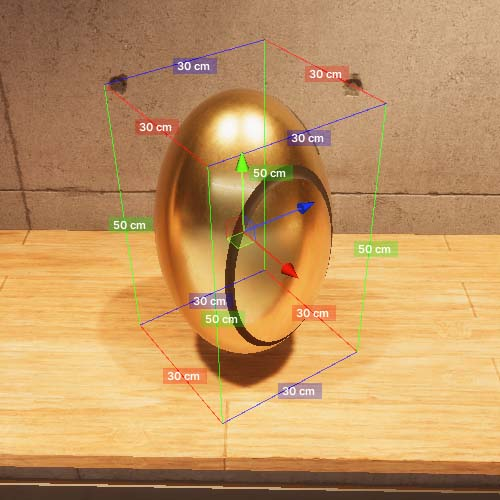Unit Selection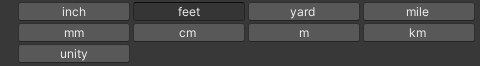All size units defined by Real Size. Custom units can be added in Preferences.

Unit Unit Description
unity The object size in Unity world space units. The calculated object size will not change when “Meters Per Unity Unit” is modified in Preferences.

Bounds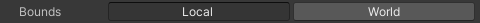Determines how an object’s bounds are calculated

Local World
Bounds are calculated relative to the selected object’s rotation Bounds are calculated relative to the world. Notice that the bounds do not rotate with the object. Each axis aligns with the world axes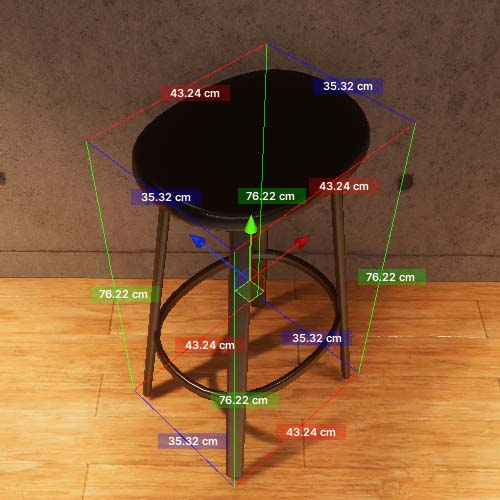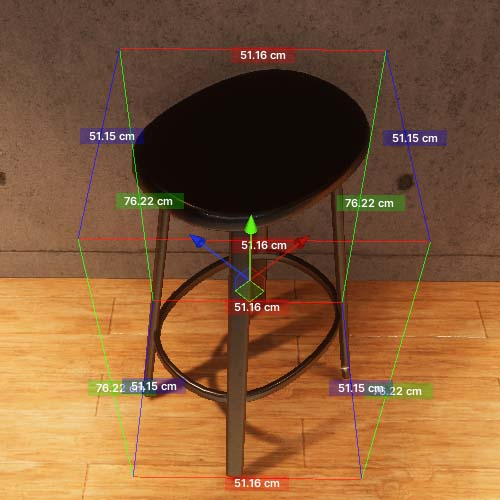Precision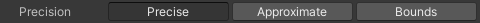Determines how precise the calculated bounds are

Precision Mode Description
Precise Calculates bounds by evaluating every vertex in the selected object. This results in the most precise bounds, but may be slow if the mesh has many (more than 150,000) vertices.
Approximate Calculates bounds by evaluating vertices, but does so by limiting the amount of vertices calculated. The number of vertices calculated is determined by the “Max Vertices Approximate Mode” setting in Preferences. The resulting bounds will not be as precise as “Precise” mode.
Bounds Calculates bounds by encapsulating the local mesh bounds of the selected object and its children. This is very fast, but the resulting bounds can be quite imprecise. This imprecision is especially apparent when the selected object is rotated, and the Bounds mode is set to “World.”

Children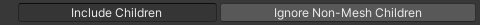Determines if and what kinds of children are included when calculating bounds

Children Toggle Description
Include Children Include child objects of the selected object when calculating bounds. Otherwise, isolate the mesh on the selected object when calculating bounds.
Ignore Non-Mesh Children Children that do not contain mesh components are not included when calculating bounds. Otherwise, objects that do not contain meshes have their Transform position encapsulated in the calculated bounds.

Draw Toggles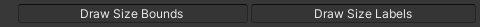Draw Toggle Description
Draw Size Bounds Draw the bounds in Scene View
Draw Size Labels Draw size labels for the bounds in Scene View

Preferences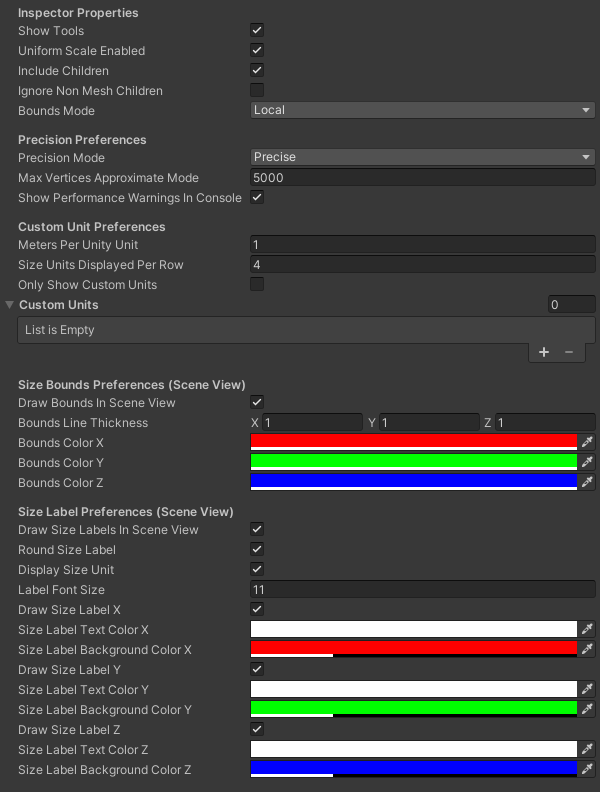Default Real Size preferences

Inspector Properties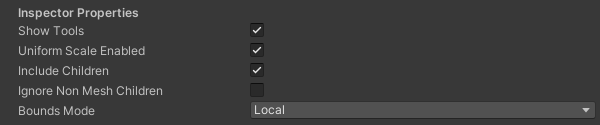All Inspector Properties are exposed in the Transform component inspector.

Precision Preferences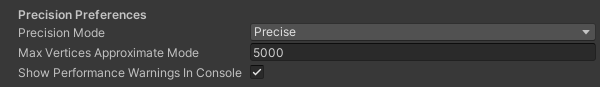Precision Preference Description
Precision Mode Determines how precise the calculated bounds of the selected object are. This property is exposed in the Transform component inspector.
Max Vertices Approximate Mode Determines the maximum number of vertices to evaluate when determining the bounds of an object when the Precision mode is set to “Approximate.” If a mesh has less vertices than the given max, all vertices will be evaluated.
Show Performance Warning In Console If calculating an object’s bounds takes longer than 20 milliseconds, a warning will appear in the Console window.

Custom Unit Preferences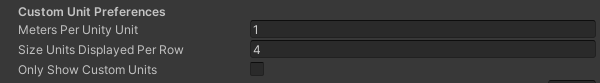Custom Unit Preference Description
Meters Per Unity Unit The amount of meters per Unity Worldspace Unit. This defaults to 1 as many physics systems assume this unit size. Setting this to a larger number will result in the calculated bounds of objects getting larger as well.
Size Units Displayed Per Row

The number of units to display per line in the Transform component inspector.

4 units per row: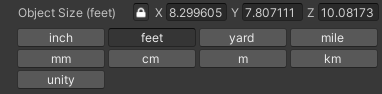8 units per row: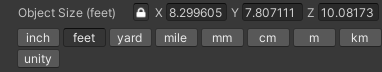Only Show Custom Units

Only show custom units defined in Preferences in the Transform component inspector. Hides the default units. However, the “unity” unit is always visible in the inspector.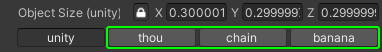Defining a Custom Unit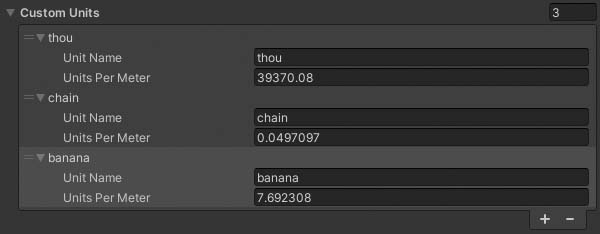Custom Unit Property Description
Unit Name The name of the unit. This will be selectable in the Transform component inspector and visible on size labels in the Scene view.
Units Per Meter

The number of units that fit within 1 meter. If you only have the Meters Per Unit of a custom unit, simply divide 1 by the Meters Per Unit measurement.

(1 / Meters Per Unit) = Units Per Meter

Example:

1 Fathom = 1.8288 Meters

1 / 1.8288 = 0.54681

Fathom Units Per Meter = 0.54681

Custom Units are selectable in the Transform component inspector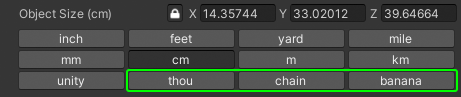Size Bound Preferences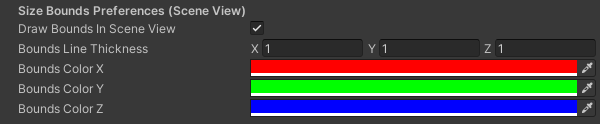Size Bound Property Description
Draw Bounds In Scene View Bounds are visible in the Scene view. This setting is exposed in the Transform component inspector.
Bounds Line Thickness The thickness of the bounds lines. Setting this to 0 will disable drawing the bounds lines for that axis.
Bounds Color (X, Y, Z) The color of the bounds lines for each axis

Size Label Preferences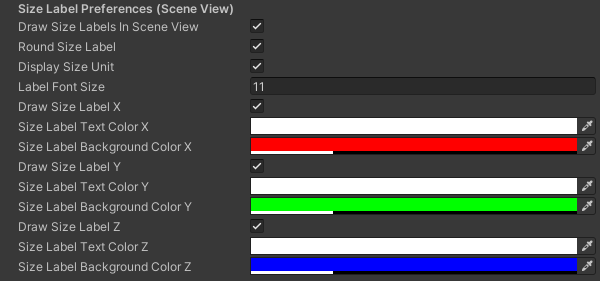Size Label Property Description
Draw Size Labels In Scene View Size Labels are visible in the Scene view. This setting is togglable in the Transform component inspector.
Round Size Label Rounds the object size to two decimal places for better visibility in the Scene view. Does not actually change the object’s size.
Display Size Unit Include the size unit after size values in size labels. If disabled, size labels only include the size values. The “unity” unit will never be included in size labels.
Label Font Size Font size for size labels
Draw Size Label (X, Y, Z) Selectively enable/disable each axis’ size label
Size Label Text Color (X, Y, Z) Text color for each axis’ size label text
Size Label Background Color (X, Y, Z) Background color for each axis’ size label

# Support

Need help?

© 2022 Real Size. Developed by Keith Swanger.